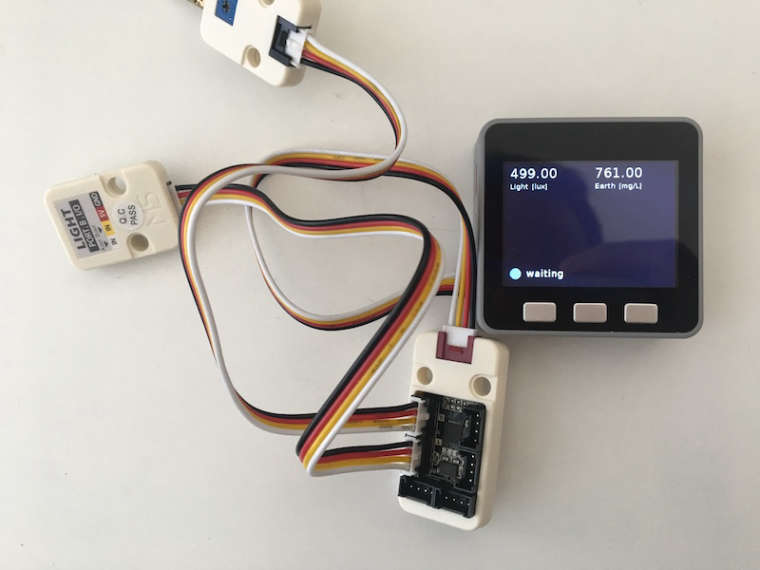# Connect units to PbHUB

• I am trying to connect a `LIGHT` and an `EARTH` unit to the `PbHUB`. I connected the `PbHUB` to `PortA`, the `EARTH` sensor to `Hub 0` and the `LIGHT` sensor to `Hub 1`.My code looks like this:

``````pbhub = unit.get(unit.PBHUB, unit.PORTA)
``````

I am able to read values from both sensors, however with strange behaviours. E.g. the lux value increases when it gets darker and vice versa. I guess the problem is, that I am lacking the logic of the `analogRead` function in the `LIGHT` and `EARTH` units:
https://github.com/m5stack/UIFlow-Code/blob/a0dbef98fe29323d6b1837b479764fdab50c3836/units/_light.py
https://github.com/m5stack/UIFlow-Code/blob/a0dbef98fe29323d6b1837b479764fdab50c3836/units/_earth.py

However I do not see how I would have to connect this unit correctly to the `PbHUB`. Do I have to copy paste the `analogRead` logic or is there another way to connect these units?
@ajb2k3 Do you know how this should be handled?

• Hey I made a video on the PBHUB https://www.youtube.com/watch?v=NG9SBKfxX5w maybe it can help you. Often when I want to write some micropython code I make it in Uiflow first. Since the micropython documentation is lacking this is a good way to find out what might be missing from your code and what the micropython commands you need are.

• @maechler You caught me here at a loss. Check out @lukasmaximus video on youtube.

• @lukasmaximus @ajb2k3 Thanks for your support! Although the video does not really answer my question.

I am able to read values from the PbHUB like this:

``````pbhub = unit.get(unit.PBHUB, unit.PORTA)
``````

The problem is, that these values seem to be wrong. The lux value increases when it gets darker, similarly the earth unit says the earth gets dryer when it actually gets wetter. I think the problem is, that reading directly from `PbHUB`, the logic from the `LIGHT.analogValue` function is missing:

``````class Light:
@property
def analogValue(self):
data = 0
max = 0
min = 4096
for i in range(0, 10):
data += newdata
if newdata > max:
max = newdata
if newdata < min:
min = newdata
data -= (max + min)
data >>= 3
return round(1024 * data / 4095, 2)
``````

This function seems to transform the data in a way that is missing when reading the value directly from `PbHUB`. My question is whether I do have to copy paste this logic or whether there is some way to reuse the `LIGHT` unit with `PbHUB`?

I would like to be able to do something like this:

``````pbhub = unit.get(unit.PBHUB, unit.PORTA)
earth = unit.get(unit.EARTH, pbhub.HUB0) # Not M5Stack API yet
light = unit.get(unit.LIGHT, pbhub.HUB1) # Not M5Stack API yet

# This should read the value correctly, using the `LIGHT.analogValue` logic
print(earth.analogValue())
print(light.analogValue())
``````

• I think the Python code in these units was not implemented with PbHUB in mind at all. I agree with your suggested interface will be very useful. You can probably do this by deriving a `HubAwareLight` class from `Light`. The job of `HubAwareLight` is to just set `newdata` value in `Light`

• @maechler are you saying the values are inverted?

• @ws1088 Thanks for the clarification!
Do you say that the value I get with `pbhub.analogRead(0x00)` should be plugged in for `newdata` in `Light.analogValue`?
I would have placed it where the Light unit calls `self.adc.readraw()`.
Do I also have to call `pbhub.analogRead(0x00)` 10 times in a loop, as the Light unit does?

@ajb2k3 Yes, kind of. I have tested what the Light unit does for increasing values of `self.adc.readraw()` it will output decreasing values. This inversion is missing when reading directly from `PbHUB`.

``````class Light:
@staticmethod
def analogValue(value):
data = 0
max = 0
min = 4096
for i in range(0, 10):
newdata = 4095 - value
data += newdata
if newdata > max:
max = newdata
if newdata < min:
min = newdata
data -= (max + min)
data >>= 3

return round(1024 * data / 4095, 2)

print(400, Light.analogValue(400))  # 400 923.98
print(500, Light.analogValue(500))  # 500 898.97
print(600, Light.analogValue(600))  # 600 873.96
print(700, Light.analogValue(700))  # 700 848.96
print(800, Light.analogValue(800))  # 800 823.95
print(900, Light.analogValue(900))  # 900 798.95
``````

• I ended up copy pasting the method from the `Light` unit with some slight adjustments:

``````def pbhubAnalogRead(pbhub, pbhub_address):
data = 0
max_val = 30
min_val = 750
for i in range(0, 10):
data += newdata
if newdata > max_val:
max_val = newdata
if newdata < min_val:
min_val = newdata
data -= (max_val + min_val)
data >>= 3
return round(max(1024 * data / 750, 0), 2)

pbhub = unit.get(unit.PBHUB, unit.PORTA)
The magic number `min_val` I got by pressing my finger on the light sensor (blocking all the light from entering) and reading the analog value. The value `750` seems to be the minimum value the light sensor would emit. The number `max_val` I got by pointing a strong light at the sensor, it seems to be the maximum value the sensor emits. The min max values are mixed up because it measures the resistance and decreasing resistance means increasing light intensity. Using this method I get values that are somewhat similar to what I get by connecting the `Light` unit to `PortB` directly.